# What is an activity 1

The activity or the thermodynamic activity is a concentration-dependent quantity that is important in physical chemistry and is given in the unit mol / l. The activity represents a kind of effective concentration that includes the effects of the non-ideality of the phase concerned.

Mathematically applies to the activity a generally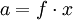It is x the mole fraction of the substance. f is a dimensionless correction factor, the activity coefficient. Gilbert Newton Lewis introduced this in 1907 as a purely empirical quantity for strong electrolytes. His theory was only physically supported by calculations by Debye and Hückel.

The activity plays an important role in the calculation of reaction rates and energy quantities of a chemical reaction (see chemical potential).

### Engineering area

In the technical area, among others, NRTL, UNIQUAC and UNIFAC are used to estimate the activity coefficient.

### Electrochemistry

According to the interpretation of Debye and Hückel is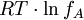the electrostatic potential energy that arises when one charges 1 mole of the ion type A from the fictitious uncharged state to its real amount of charge within its oppositely charged ion cloud. This potential energy is therefore negative ("You have to put energy into it in order to remove the ion type A from your ion cloud").

Debye-Hückel: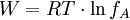In it is R. the universal gas constant and T the temperature. W is negative; thus f lies between 0 and 1. (Debye-Hückel's theory requires a strong dilution of A)

Experimental measurements revealed a relationship between the ionic concentration of A and its activity coefficient. For large concentrations of A, f becomes greater than one ("I gain energy when I remove A"). Large ion concentrations of A are not taken into account by the Debye-Hückel theory.

Example: For 1 molar acetic acid is f for example 0.8, but already 0.96 for 0.1 molar acetic acid.

### Activity of a solvent

In solutions there is activity aB. of a substance B. defined by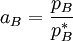in which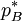is the vapor pressure of the pure solvent (which is chosen as the standard fugacity), pB. the vapor pressure of the solvent in the solution (actually the fugacity). In this case, a is to be seen analogously to the mole fraction: This is the mole fraction, which is decisive when considering the system. Raoult's law applies to solvents all the more, the closer the mole fraction approaches 1, i.e. the purer the solvent. In order to clearly describe the behavior, a correction factor has also been introduced here, which is now aB. defined as follows:

aB. = γB.xB. With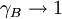For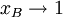This relationship can be derived from the chemical potential and Raoult's law.

#### Marginal activity coefficient

The marginal activity coefficient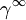is the activity coefficient of a component at infinite dilution (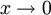) in a solvent or a mixture of solvents.

### Activity of ions and electrolytes

For dilute electrolytes, the activity coefficient can be calculated from the Debye-Hückel theory. It should be noted that the definition equation given above only applies to each individual type of ion i applies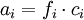However, since every solution has to be electroneutral, i.e. it has to have the same number of positive and negative charges, individual activities can be calculated usefully, but not measured. For one according to the equation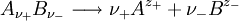fully dissociating electrolyte applies if we use the indices + for the cations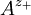and - for the anions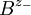use,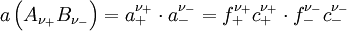To simplify it will be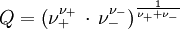the medium activity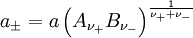and the mean activity coefficient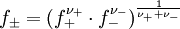Are defined.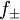is z. B. measurable in galvanic cells. The following then applies to the mean activity of the electrolyte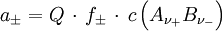In it is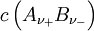the concentration of the electrolyte.

Examples:

For an aqueous solution that contains 10-3 mol dm-3 KCl and Na2SO4 contains, becomes from the Debye-Hückel limit law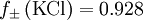calculated. It is Q = 1, so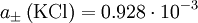mol dm-3.
For 0.1 mol dm-3 MgCl2 is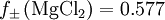. With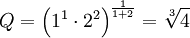is so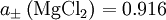mol dm-3.